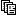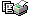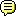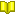Forum Index | Search | Register (New User) | Login (Existing User) | Who's Online | FAQ | Main Site

Register (or login) on our website and you will not see this ad.Pages in this thread: 1 | 2 | 3 | 4 |  | (show all)Print Threadngllgn(newbie) Sat 12-Jan-13 22:38:35## Re: Use 3com router 3crwdr100y-72 as wifi signal amplifier

In reply to a post by yarwell:
I meant the phone connection provided on the router. Is there any way, with admin privileges, to reconfigure the part of the default configuration that Vodafone imposes, but that the router does not receive automatically?
If you can locate the settings page(s) for VOIP or SIP on the router interface, and find the relevant settings to use on the net it should be practical.

I can locate and identify the VOIP and SIP settings on the router interface, but only when in admin mode. And of course when in admin mode I can also save a back-up copy of the settings. The problem is that when I am in admin mode, the telephony service is disabled and so even if I have the back-up to look at as a text file, the telephony settings probably won't be correct. In fact I tried this but I am too ignorant to know what the admin mode settings mean, never mind what they should be to make telephony work. The best bet is probably to try and find them on the net, as you say. I'll have a trawl around tomorrow.

Here's a thought - can you let the vodafone settings be installed and then save them to a file from the cut-down user interface ? if so you could load them up manually by reading what's in the file.

No. The cut-down user interface will not let me back up the configuration file.ngllgn(newbie) Sun 13-Jan-13 14:31:35## Re: Use 3com router 3crwdr100y-72 as wifi signal amplifier

¿Might there be some information in this back-up I have made of the configuration file when in admin mode that would give a clue to the incorrect telefony settings?

<psitree>
<WirelessCfg>
<vars state="enabled" curState="enabled" ssIdIndex="0" country="ES" ses_enable="1" ses_event="2" ses_states="01:01" ses_ssid="" ses_hide="0" ses_wpa_psk="" ses_auth="0" ses_auth_mode="" ses_wep="" ses_wpa="" ses_client_enable="1" ses_client_event="0" ses_wds_mode="2" ses_wds_wsec="" apMode="ap" bridgeRestrict="disabled" wdsMAC_0="" wdsMAC_1="" wdsMAC_2="" wdsMAC_3="" band="b" channel="0" rate="auto" multicastRate="auto" basicRate="default" fragThreshold="2346" RTSThreshold="2346" DTIM="1" beacon="100" XPress="off" gMode="auto" gProtection="auto" preamble="long" AfterBurner="off" TxPowerPercent="100" WME="on" WMENoAck="off" WMEApsd="on" RadioCtl="on" RegulatoryMode="off" PreNetRadarDectTime="60" InNetRadarDectTime="60" TpcMitigation="0" AutoChannelTimer="0" globalMaxAssoc="128" AutoSwOffdays="0" StarOfftTime="0" EndOffTime="0" NBandwidthCap="1" NCtrlSideband="lower" NBand="2" NMCSIdx="-1" NProtection="auto" NMode="auto" NReqd="off" />
<wlMssidVars tableSize="4">
<wlMssidEntry enblSsId="1" ssId="vodafone78A2" hide="0" apIsolation="off" fltMacMode="disabled" disableWme="off" MaxAssoc = "32" wsc_mode="enabled" wsc_config_state="1" authMode="psk" radiusServerIP="0.0.0.0" radiusServerPort="1812" radiusServerKey="" wep="disabled" auth="0" keyBit="128-bit" key64_1="" key64_2="" key64_3="" key64_4="" key64Index="1" key128_1="1234567890123" key128_2="" key128_3="" key128_4="" key128Index="1" wpaRekey="0" wpakey="PKQKAKUJAQTWRR" Preauthentication="off" ReauthTimeout="36000" wpa="aes" tr69cBeaconType="Basic" tr69cBasicEncryptionModes="None" tr69cBasicAuthenticationMode="None" tr69cWPAEncryptionModes="WEPEncryption" tr69cWPAAuthenticationMode="PSKAuthentication" tr69cIEEE11iEncryptionModes="AESEncryption" tr69cIEEE11iAuthenticationMode="PSKAuthentication"/>
<wlMssidEntry enblSsId="0" ssId="vodafone78A2-Guest" hide="0" apIsolation="off" fltMacMode="disabled" disableWme="off" MaxAssoc = "32" wsc_mode="disabled" wsc_config_state="1" authMode="psk2mix" radiusServerIP="0.0.0.0" radiusServerPort="1812" radiusServerKey="" wep="disabled" auth="0" keyBit="128-bit" key64_1="" key64_2="" key64_3="" key64_4="" key64Index="1" key128_1="" key128_2="" key128_3="" key128_4="" key128Index="1" wpaRekey="0" wpakey="PKQKAKUJAQTWRR" Preauthentication="off" ReauthTimeout="36000" wpa="aes" tr69cBeaconType="Basic" tr69cBasicEncryptionModes="None" tr69cBasicAuthenticationMode="None" tr69cWPAEncryptionModes="WEPEncryption" tr69cWPAAuthenticationMode="PSKAuthentication" tr69cIEEE11iEncryptionModes="AESEncryption" tr69cIEEE11iAuthenticationMode="PSKAuthentication"/>
<wlMssidEntry enblSsId="0" ssId="Guest1" hide="0" apIsolation="off" fltMacMode="disabled" disableWme="off" MaxAssoc = "32" wsc_mode="disabled" wsc_config_state="1" authMode="open" radiusServerIP="0.0.0.0" radiusServerPort="1812" radiusServerKey="" wep="disabled" auth="0" keyBit="128-bit" key64_1="" key64_2="" key64_3="" key64_4="" key64Index="1" key128_1="" key128_2="" key128_3="" key128_4="" key128Index="1" wpaRekey="0" wpakey="" Preauthentication="off" ReauthTimeout="36000" wpa="aes" tr69cBeaconType="Basic" tr69cBasicEncryptionModes="None" tr69cBasicAuthenticationMode="None" tr69cWPAEncryptionModes="WEPEncryption" tr69cWPAAuthenticationMode="PSKAuthentication" tr69cIEEE11iEncryptionModes="AESEncryption" tr69cIEEE11iAuthenticationMode="PSKAuthentication"/>
<wlMssidEntry enblSsId="0" ssId="Guest2" hide="0" apIsolation="off" fltMacMode="disabled" disableWme="off" MaxAssoc = "32" wsc_mode="disabled" wsc_config_state="1" authMode="open" radiusServerIP="0.0.0.0" radiusServerPort="1812" radiusServerKey="" wep="disabled" auth="0" keyBit="128-bit" key64_1="" key64_2="" key64_3="" key64_4="" key64Index="1" key128_1="" key128_2="" key128_3="" key128_4="" key128Index="1" wpaRekey="0" wpakey="" Preauthentication="off" ReauthTimeout="36000" wpa="aes" tr69cBeaconType="Basic" tr69cBasicEncryptionModes="None" tr69cBasicAuthenticationMode="None" tr69cWPAEncryptionModes="WEPEncryption" tr69cWPAAuthenticationMode="PSKAuthentication" tr69cIEEE11iEncryptionModes="AESEncryption" tr69cIEEE11iAuthenticationMode="PSKAuthentication"/>
</wlMssidVars>
</WirelessCfg>
<E220>
<Entry LinkMode="0" OperationMode="0" ConType="1" Channel="0" VoiceChannel ="1" VoiceEnable ="1" VoiceInterface="0"/>
</E220>
<TR143Cfg>
<UploadDiagnostics DiagnosticsState="None" Interface="" UploadURL="" DSCP="0" EthernetPriority="0" TestFileLength="0"/>
<UDPEchoConfig Enable="0" Interface="" SourceIPAddress ="" UDPPort="0" EchoPlusEnabled="0"/>
</TR143Cfg>
<Hspacfg>
</Hspacfg>
<SystemInfo>
<protocol autoScan="enable" igmpSnp ="disable" macFilterPolicy="forward" siproxd="disable" ddns="disable"/>
<sysLog state="enable" displayLevel="CRIT" logLevel="CRIT" option="local" serverIP="0.0.0.0" serverPort="514" fwlog="disable" Syslog="disable"/>
<sysHttp port="80"/>
<siproxd enable="disable" port="5060"/>
<snmp state="enable" readCommunity="s0l0tuya" writeCommunity="4rEAd&wrItE" sysName="unknown" sysLocation="unknown" sysContact="unknown" trapIP="0.0.0.0" debug="0" configId="00000000"/>
<upnpvideo service="enable" provision="enable"/>
</SystemInfo>
<AtmCfg>
<initCfg structureId="2" threadPriority="25" freeCellQSize="10" freePktQSize="200" freePktQBufSize="1600" freePktQBufOffset="32" rxCellQSize="10" rxPktQSize="200" txFifoPriority="64" aal5MaxSduLen="64" aal2MaxSduLen="0"/>
</AtmCfg>
<AtmCfgTd>
<td1 cat="UBR" PCR="0" SCR="0" MBS="0"/>
<td2 cat="CBR" PCR="579" SCR="0" MBS="0"/>
</AtmCfgTd>
<AtmCfgVcc>
<vccId9999 vpi="0" vci="65534" tdId="0" aalType="AAL2" adminStatus="down" encap="unknown" qos="disable" instanceId="1509949444"/>
<vccId1 vpi="255" vci="65535" tdId="1" aalType="AAL5" adminStatus="up" encap="unknown" qos="enable" instanceId="1509949441"/>
<vccId2 vpi="0" vci="44" tdId="1" aalType="AAL5" adminStatus="up" encap="llc" qos="enable" instanceId="1509949442"/>
<vccId3 vpi="0" vci="34" tdId="2" aalType="AAL5" adminStatus="up" encap="llc" qos="disable" instanceId="1509949443"/>
<vccId4 vpi="0" vci="33" tdId="1" aalType="AAL5" adminStatus="up" encap="llc" qos="enable" instanceId="1509949444"/>
</AtmCfgVcc>
<SecCfg>
<srvCtrlList ftp="lan" http="enable" icmp="enable" snmp="wan" ssh="disable" telnet="enable" tftp="disable"/>
<qosService tableSize="52">
<qosServiceEntry id="1" parent="1" serviceName="HTTP" protocol="6" srcPort="80" srcMaxPort="" dstPort="" dstMaxPort=""/>
<qosServiceEntry id="2" parent="1" serviceName="HTTP" protocol="6" srcPort="" srcMaxPort="" dstPort="80" dstMaxPort=""/>
<qosServiceEntry id="1" parent="2" serviceName="HTTPs" protocol="6" srcPort="443" srcMaxPort="" dstPort="" dstMaxPort=""/>
<qosServiceEntry id="2" parent="2" serviceName="HTTPs" protocol="6" srcPort="" srcMaxPort="" dstPort="443" dstMaxPort=""/>
<qosServiceEntry id="1" parent="3" serviceName="FTP" protocol="6" srcPort="21" srcMaxPort="" dstPort="" dstMaxPort=""/>
<qosServiceEntry id="2" parent="3" serviceName="FTP" protocol="6" srcPort="" srcMaxPort="" dstPort="21" dstMaxPort=""/>
<qosServiceEntry id="3" parent="3" serviceName="FTP" protocol="6" srcPort="20" srcMaxPort="" dstPort="" dstMaxPort=""/>
<qosServiceEntry id="4" parent="3" serviceName="FTP" protocol="6" srcPort="" srcMaxPort="" dstPort="20" dstMaxPort=""/>
<qosServiceEntry id="1" parent="4" serviceName="RIP" protocol="17" srcPort="520" srcMaxPort="" dstPort="" dstMaxPort=""/>
<qosServiceEntry id="1" parent="5" serviceName="DNS" protocol="6" srcPort="53" srcMaxPort="" dstPort="" dstMaxPort=""/>
<qosServiceEntry id="2" parent="5" serviceName="DNS" protocol="6" srcPort="" srcMaxPort="" dstPort="53" dstMaxPort=""/>
<qosServiceEntry id="3" parent="5" serviceName="DNS" protocol="17" srcPort="53" srcMaxPort="" dstPort="" dstMaxPort=""/>
<qosServiceEntry id="4" parent="5" serviceName="DNS" protocol="17" srcPort="" srcMaxPort="" dstPort="53" dstMaxPort=""/>
<qosServiceEntry id="1" parent="6" serviceName="DHCP" protocol="17" srcPort="" srcMaxPort="" dstPort="67" dstMaxPort="68"/>
<qosServiceEntry id="1" parent="7" serviceName="ICMP" protocol="1" srcPort="" srcMaxPort="" dstPort="" dstMaxPort=""/>
<qosServiceEntry id="1" parent="8" serviceName="IGMP" protocol="2" srcPort="" srcMaxPort="" dstPort="" dstMaxPort=""/>
<qosServiceEntry id="1" parent="9" serviceName="Telnet" protocol="6" srcPort="23" srcMaxPort="" dstPort="" dstMaxPort=""/>
<qosServiceEntry id="2" parent="9" serviceName="Telnet" protocol="6" srcPort="" srcMaxPort="" dstPort="23" dstMaxPort=""/>
<qosServiceEntry id="1" parent="10" serviceName="HTTP Proxy" protocol="6" srcPort="8080" srcMaxPort="" dstPort="" dstMaxPort=""/>
<qosServiceEntry id="2" parent="10" serviceName="HTTP Proxy" protocol="6" srcPort="" srcMaxPort="" dstPort="8080" dstMaxPort=""/>
<qosServiceEntry id="1" parent="11" serviceName="POP2" protocol="6" srcPort="109" srcMaxPort="" dstPort="" dstMaxPort=""/>
<qosServiceEntry id="2" parent="11" serviceName="POP2" protocol="6" srcPort="" srcMaxPort="" dstPort="109" dstMaxPort=""/>
<qosServiceEntry id="1" parent="12" serviceName="POP3" protocol="6" srcPort="110" srcMaxPort="" dstPort="" dstMaxPort=""/>
<qosServiceEntry id="2" parent="12" serviceName="POP3" protocol="6" srcPort="" srcMaxPort="" dstPort="110" dstMaxPort=""/>
<qosServiceEntry id="3" parent="12" serviceName="POP3" protocol="17" srcPort="110" srcMaxPort="" dstPort="" dstMaxPort=""/>
<qosServiceEntry id="4" parent="12" serviceName="POP3" protocol="17" srcPort="" srcMaxPort="" dstPort="110" dstMaxPort=""/>
<qosServiceEntry id="1" parent="13" serviceName="POP3s" protocol="6" srcPort="995" srcMaxPort="" dstPort="" dstMaxPort=""/>
<qosServiceEntry id="2" parent="13" serviceName="POP3s" protocol="6" srcPort="" srcMaxPort="" dstPort="995" dstMaxPort=""/>
<qosServiceEntry id="3" parent="13" serviceName="POP3s" protocol="17" srcPort="995" srcMaxPort="" dstPort="" dstMaxPort=""/>
<qosServiceEntry id="4" parent="13" serviceName="POP3s" protocol="17" srcPort="" srcMaxPort="" dstPort="995" dstMaxPort=""/>
<qosServiceEntry id="1" parent="14" serviceName="SMTP" protocol="6" srcPort="25" srcMaxPort="" dstPort="" dstMaxPort=""/>
<qosServiceEntry id="2" parent="14" serviceName="SMTP" protocol="6" srcPort="" srcMaxPort="" dstPort="25" dstMaxPort=""/>
<qosServiceEntry id="1" parent="15" serviceName="IMAPS" protocol="6" srcPort="993" srcMaxPort="" dstPort="" dstMaxPort=""/>
<qosServiceEntry id="2" parent="15" serviceName="IMAPS" protocol="6" srcPort="" srcMaxPort="" dstPort="993" dstMaxPort=""/>
<qosServiceEntry id="1" parent="16" serviceName="SSH" protocol="6" srcPort="22" srcMaxPort="" dstPort="" dstMaxPort=""/>
<qosServiceEntry id="2" parent="16" serviceName="SSH" protocol="6" srcPort="" srcMaxPort="" dstPort="22" dstMaxPort=""/>
<qosServiceEntry id="1" parent="17" serviceName="IPSec" protocol="17" srcPort="500" srcMaxPort="" dstPort="" dstMaxPort=""/>
<qosServiceEntry id="2" parent="17" serviceName="IPSec" protocol="17" srcPort="" srcMaxPort="" dstPort="500" dstMaxPort=""/>
<qosServiceEntry id="3" parent="17" serviceName="IPSec" protocol="50" srcPort="" srcMaxPort="" dstPort="" dstMaxPort=""/>
<qosServiceEntry id="4" parent="17" serviceName="IPSec" protocol="51" srcPort="" srcMaxPort="" dstPort="" dstMaxPort=""/>
<qosServiceEntry id="1" parent="18" serviceName="SIP" protocol="6" srcPort="5060" srcMaxPort="" dstPort="" dstMaxPort=""/>
<qosServiceEntry id="2" parent="18" serviceName="SIP" protocol="6" srcPort="" srcMaxPort="" dstPort="5060" dstMaxPort=""/>
<qosServiceEntry id="3" parent="18" serviceName="SIP" protocol="17" srcPort="5060" srcMaxPort="" dstPort="" dstMaxPort=""/>
<qosServiceEntry id="4" parent="18" serviceName="SIP" protocol="17" srcPort="" srcMaxPort="" dstPort="5060" dstMaxPort=""/>
<qosServiceEntry id="1" parent="19" serviceName="RTP" protocol="17" srcPort="50000" srcMaxPort="50020" dstPort="" dstMaxPort=""/>
<qosServiceEntry id="1" parent="20" serviceName="RTCP" protocol="17" srcPort="50000" srcMaxPort="50020" dstPort="" dstMaxPort=""/>
<qosServiceEntry id="1" parent="21" serviceName="SNMP" protocol="17" srcPort="161" srcMaxPort="162" dstPort="" dstMaxPort=""/>
<qosServiceEntry id="2" parent="21" serviceName="SNMP" protocol="17" srcPort="" srcMaxPort="" dstPort="161" dstMaxPort="162"/>
<qosServiceEntry id="1" parent="22" serviceName="SSMTP" protocol="6" srcPort="465" srcMaxPort="" dstPort="" dstMaxPort=""/>
<qosServiceEntry id="2" parent="22" serviceName="SSMTP" protocol="6" srcPort="" srcMaxPort="" dstPort="465" dstMaxPort=""/>
<qosServiceEntry id="3" parent="22" serviceName="SSMTP" protocol="17" srcPort="465" srcMaxPort="" dstPort="" dstMaxPort=""/>
<qosServiceEntry id="4" parent="22" serviceName="SSMTP" protocol="17" srcPort="" srcMaxPort="" dstPort="465" dstMaxPort=""/>
</qosService>
<accCtrlMode state="enable"/>
<secDosCfg enable="1" syn="0" fin="0" udp="0" icmp="0" synPerIp="0" finPerIp="0" udpPerIp="0" icmpPerIp="0" portScan="0" icmpSmurf="0" ipLand="1" SameSrcDst="1" ipSproof="1" ipTeardrop="1" pingOfDeath="1" tcpScan="0" SynWithData="0" udpBomb="0" ptScanSens="0" synSpd="50" finSpd="50" udpSpd="500" icmpSpd="10" synPerIpSpd="10" finPerIpSpd="10" udpPerIpSpd="100" icmpPerIpSpd="5" BrdcstSrc="1" LanSrcIp="1" Frgmthdr="1" InvalidTcpFlg="1"/>
<secFwCfg level="disable"/>
<qosQueue tableSize="6">
<qosQueueEntry id="1" precedence="1" weight="0" mark="1" schAlg="SP"/>
<qosQueueEntry id="2" precedence="2" weight="0" mark="2" schAlg="WRR"/>
<qosQueueEntry id="3" precedence="2" weight="0" mark="3" schAlg="WRR"/>
<qosQueueEntry id="4" precedence="3" weight="0" mark="4" schAlg="SP"/>
<qosQueueEntry id="5" precedence="2" weight="0" mark="5" schAlg="WRR"/>
<qosQueueEntry id="6" precedence="2" weight="0" mark="6" schAlg="WRR"/>
</qosQueue>
<qosCfg enable="1"/>
<secEnblCfg fw_enable="0" inflt_enable="0" outflt_enable="0" macflt_enable="0" macmngr_enable="0" nat_enable="1"/>
<accCtrl tableSize="8">
</accCtrl>
<qosCls tableSize="10">
</qosCls>
</SecCfg>
<Lan>
<entry9999 address="1.1.1.1" mask="255.255.255.0" dhcpServer="disable" dhcpRelay="0" leasedTime="0" startAddr="0.0.0.0" endAddr="0.0.0.0" reservedAddr="" dhcpNtpServer1="0.0.0.0" dhcpNtpServer2="0.0.0.0" lanDns1="0.0.0.0" lanDns2="0.0.0.0" opt43="" opt66="" opt67="" opt160="" lanDomain="" dhcpClassId="" instanceId="1509949445" addrType="3"/>
<entry1 address="192.168.0.1" mask="255.255.255.0" dhcpServer="enable" dhcpRelay="0" leasedTime="24" startAddr="192.168.0.192" endAddr="192.168.0.254" reservedAddr="" dhcpNtpServer1="0.0.0.0" dhcpNtpServer2="0.0.0.0" lanDns1="192.168.0.1" lanDns2="192.168.0.1" opt43="" opt66="" opt67="" opt160="" lanDomain="Vodafone.DSLRouter" dhcpClassId="" instanceId="1509949441" addrType="2"/>
<ethCfg1024 speed="auto" type="auto" MTU="1500" disabled="0"/>
<ethCfg1025 speed="auto" type="auto" MTU="1500" disabled="0"/>
<ethCfg1026 speed="auto" type="auto" MTU="1500" disabled="0"/>
<ethCfg1027 speed="auto" type="auto" MTU="1500" disabled="0"/>
</Lan>
<RouteCfg>
<ripGlobal state="disable" ripIfcTableSize="1"/>
<ripIfc tableSize="1">
<ripIfcEntry id="1" name="br0" state="disable" version="2" operation="active"/>
</ripIfc>
</RouteCfg>
<PMapCfg>
<pmap tableSize="1">
<pmapEntry id="1" groupName="Default" ifList="ath0|eth0" vendorid0="" vendorid1="" vendorid2="" vendorid3="" vendorid4=""/>
</pmap>
<pmapIfcCfg pmapIfName="eth0" pmapIfcStatus="disable"/>
</PMapCfg>
<SambaCfg>
<sambaStatus enableSamba="enable" workgrp="vodafone" svrname="vodafone" netbname="vodafone"/>
</SambaCfg>
<Voice>
<sip_phonecfg speak="0" listen="0" interval="50" region="14" minHookFlash="80" maxHookFlash="250" digitmap="[X*#ABCD].T|9XXXXXXXX|8XXXXXXXX|7XXXXXXXX|6XXXXXXXX" offhooktime="250" onhooktime="250" fstdialtime="150" autocallenbl="0" autocallnum="" autocallinterval="70" reinjection="0" innercall="1" modemservice="1"/>
<sipAdvanced sipSrvRegPeriod="1800" sipSrvExpire="3600" sipSrvRegister="10" sipSrvSessionExpires="32000" sipSrvMinSe="90" sipMaxRetryTime="32" sipPrimaryCheckTime="300" sipMWIExpire="3600" sipRtpStartPort="50000" sipRtpEndPort="50020" sipRtpTxReptatInterval="3000" sipRtcpMonitor="0" sipInterface="br0" sipAdvLocalPort="6050" pstnCallPrefix="UNSPT" sipTestEnabled="0" dscpmark="46" />
<sipQos sipQosTos="0" sipQosVlanEnbled="0" sipQosVlanId="0" sipQosPriority="3"/>
<sipBasic tableSize="2">
<sipBasicEntry id="1" sipUserId="" sipUserName="" sipUserPasswd="" sipLocalPort="6050" sipFwdTblId="1" sipClipEnable="1" sipLineEnable="0" sipLineCallHold="1" sipLineCallWait="1" sipLine3Way="1" sipLineCallTransfer="0" sipLineMwi="0" sipLineMwiAccount="" sipLineFaxDetect="1" vad="0" ec="1" cng="0" sipVoiceCapCodec1="8" sipVoiceCapCodec2="0" sipVoiceCapCodec3="9" sipVoiceCapCodec4="2" sipVoiceCapCodec5="18" sipVoiceCapPTime1="20" sipVoiceCapPTime2="20" sipVoiceCapPTime3="20" sipVoiceCapPTime4="20" sipVoiceCapPTime5="20" sipVoiceCapPriority1="1" sipVoiceCapPriority2="3" sipVoiceCapPriority3="5" sipVoiceCapPriority4="4" sipVoiceCapPriority5="2" sipVoiceCapIfEnable1="1" sipVoiceCapIfEnable2="1" sipVoiceCapIfEnable3="0" sipVoiceCapIfEnable4="0" sipVoiceCapIfEnable5="1" sipVoiceDtmfType="1" sipFaxType="0" sipSpNum00="" sipSpName00="" sipApNum00="" sipApName00="" sipSsNum00="" sipSsName00="" sipAsNum00="" sipAsName00="" sipSpNum01="" sipSpName01="" sipApNum01="" sipApName01="" sipSsNum01="" sipSsName01="" sipAsNum01="" sipAsName01="" sipSpNum02="" sipSpName02="" sipApNum02="" sipApName02="" sipSsNum02="" sipSsName02="" sipAsNum02="" sipAsName02="" sipSpNum03="" sipSpName03="" sipApNum03="" sipApName03="" sipSsNum03="" sipSsName03="" sipAsNum03="" sipAsName03="" sipSpNum04="" sipSpName04="" sipApNum04="" sipApName04="" sipSsNum04="" sipSsName04="" sipAsNum04="" sipAsName04="" sipSpNum05="" sipSpName05="" sipApNum05="" sipApName05="" sipSsNum05="" sipSsName05="" sipAsNum05="" sipAsName05="" sipSpNum06="" sipSpName06="" sipApNum06="" sipApName06="" sipSsNum06="" sipSsName06="" sipAsNum06="" sipAsName06="" sipSpNum07="" sipSpName07="" sipApNum07="" sipApName07="" sipSsNum07="" sipSsName07="" sipAsNum07="" sipAsName07="" sipSpNum08="" sipSpName08="" sipApNum08="" sipApName08="" sipSsNum08="" sipSsName08="" sipAsNum08="" sipAsName08="" sipSpNum09="" sipSpName09="" sipApNum09="" sipApName09="" sipSsNum09="" sipSsName09="" sipAsNum09="" sipAsName09="" sipSpNum10="" sipSpName10="" sipApNum10="" sipApName10="" sipSsNum10="" sipSsName10="" sipAsNum10="" sipAsName10="" sipSpNum11="" sipSpName11="" sipApNum11="" sipApName11="" sipSsNum11="" sipSsName11="" sipAsNum11="" sipAsName11="" sipSpNum12="" sipSpName12="" sipApNum12="" sipApName12="" sipSsNum12="" sipSsName12="" sipAsNum12="" sipAsName12="" sipSpNum13="" sipSpName13="" sipApNum13="" sipApName13="" sipSsNum13="" sipSsName13="" sipAsNum13="" sipAsName13="" sipSpNum14="" sipSpName14="" sipApNum14="" sipApName14="" sipSsNum14="" sipSsName14="" sipAsNum14="" sipAsName14="" sipSpNum15="" sipSpName15="" sipApNum15="" sipApName15="" sipSsNum15="" sipSsName15="" sipAsNum15="" sipAsName15=""/>
<sipBasicEntry id="2" sipUserId="" sipUserName="" sipUserPasswd="" sipLocalPort="6050" sipFwdTblId="1" sipClipEnable="1" sipLineEnable="0" sipLineCallHold="1" sipLineCallWait="1" sipLine3Way="1" sipLineCallTransfer="0" sipLineMwi="0" sipLineMwiAccount="" sipLineFaxDetect="1" vad="0" ec="1" cng="0" sipVoiceCapCodec1="8" sipVoiceCapCodec2="0" sipVoiceCapCodec3="9" sipVoiceCapCodec4="2" sipVoiceCapCodec5="18" sipVoiceCapPTime1="20" sipVoiceCapPTime2="20" sipVoiceCapPTime3="20" sipVoiceCapPTime4="20" sipVoiceCapPTime5="20" sipVoiceCapPriority1="1" sipVoiceCapPriority2="3" sipVoiceCapPriority3="5" sipVoiceCapPriority4="4" sipVoiceCapPriority5="2" sipVoiceCapIfEnable1="1" sipVoiceCapIfEnable2="1" sipVoiceCapIfEnable3="0" sipVoiceCapIfEnable4="0" sipVoiceCapIfEnable5="1" sipVoiceDtmfType="1" sipFaxType="0" sipSpNum00="" sipSpName00="" sipApNum00="" sipApName00="" sipSsNum00="" sipSsName00="" sipAsNum00="" sipAsName00="" sipSpNum01="" sipSpName01="" sipApNum01="" sipApName01="" sipSsNum01="" sipSsName01="" sipAsNum01="" sipAsName01="" sipSpNum02="" sipSpName02="" sipApNum02="" sipApName02="" sipSsNum02="" sipSsName02="" sipAsNum02="" sipAsName02="" sipSpNum03="" sipSpName03="" sipApNum03="" sipApName03="" sipSsNum03="" sipSsName03="" sipAsNum03="" sipAsName03="" sipSpNum04="" sipSpName04="" sipApNum04="" sipApName04="" sipSsNum04="" sipSsName04="" sipAsNum04="" sipAsName04="" sipSpNum05="" sipSpName05="" sipApNum05="" sipApName05="" sipSsNum05="" sipSsName05="" sipAsNum05="" sipAsName05="" sipSpNum06="" sipSpName06="" sipApNum06="" sipApName06="" sipSsNum06="" sipSsName06="" sipAsNum06="" sipAsName06="" sipSpNum07="" sipSpName07="" sipApNum07="" sipApName07="" sipSsNum07="" sipSsName07="" sipAsNum07="" sipAsName07="" sipSpNum08="" sipSpName08="" sipApNum08="" sipApName08="" sipSsNum08="" sipSsName08="" sipAsNum08="" sipAsName08="" sipSpNum09="" sipSpName09="" sipApNum09="" sipApName09="" sipSsNum09="" sipSsName09="" sipAsNum09="" sipAsName09="" sipSpNum10="" sipSpName10="" sipApNum10="" sipApName10="" sipSsNum10="" sipSsName10="" sipAsNum10="" sipAsName10="" sipSpNum11="" sipSpName11="" sipApNum11="" sipApName11="" sipSsNum11="" sipSsName11="" sipAsNum11="" sipAsName11="" sipSpNum12="" sipSpName12="" sipApNum12="" sipApName12="" sipSsNum12="" sipSsName12="" sipAsNum12="" sipAsName12="" sipSpNum13="" sipSpName13="" sipApNum13="" sipApName13="" sipSsNum13="" sipSsName13="" sipAsNum13="" sipAsName13="" sipSpNum14="" sipSpName14="" sipApNum14="" sipApName14="" sipSsNum14="" sipSsName14="" sipAsNum14="" sipAsName14="" sipSpNum15="" sipSpName15="" sipApNum15="" sipApName15="" sipSsNum15="" sipSsName15="" sipAsNum15="" sipAsName15=""/>
</sipBasic>
<sipProvisioned sipClipEnableProv="1" sipLineCallHoldProv="1" sipLineCallWaitProv="1" sipLine3WayProv="1" sipLineCallTransferProv="0" sipLineMwiProv="0" sipLineAutoCallProv="1"/>
<sipServer tableSize="2">
<sipServerEntry id="1" sipRegIP="" sipRegPort="5060" sipPrxyIP="" sipPrxyPort="5060" sipDomain=""/>
<sipServerEntry id="2" sipRegIP="" sipRegPort="5060" sipPrxyIP="" sipPrxyPort="5060" sipDomain=""/>
</sipServer>
</Voice>
<SNTPCfg>
<cfg state="enable" CfgServer="disable" server1="172.30.255.1" server2="87.235.0.10" timezone="Brussels, Copenhagen, Madrid, Paris" FailTime="5" SucTime="21600" EnableSum="1"/>
</SNTPCfg>
<PPPProfile>
<pppPro tableSize="1">
<pppProEntry id="1" ProName="123" PhoneNum="*99#" auth="0" autodns="0" preferredDns="255.255.255.255" alternateDns="255.255.255.255" autoApn="1"/>
</pppPro>
</PPPProfile>
<dhcphostname>
<Entry option12="Huawei-HG556a"/>
</dhcphostname>
<pppsrv_255_65535>
<ppp_conId1 serviceName="" idleTimeout="0" ipExt="disable" auth="auto" useStaticIpAddr="0" localIpAddr="0.0.0.0" Debug="disable" ProName="123" automanualConnect="1" delayRedial="25" timeout="3" delayTime="15" BridgeMixed="disable" Mtu="1492" LcpEchoInterval="30" IpExtMac="" enblpppVoB="disable" pppVoBitstreamIPAddr="0.0.0.0"/>
</pppsrv_255_65535>
<wan_255_65535>
<entry1 vccId="1" conId="1" name="pppou_255_65535_1" protocol="PPPOU" encap="LLC" firewall="enable" nat="enable" vlanId="0" service="enable" instanceId="1509949442" primary="0.0.0.0" secondary="0.0.0.0" domain="" gw="0.0.0.0"/>
</wan_255_65535>
<igmpproxy>
<proxy_node igmpEnable="0" interface="none" version="2" groups="32" logSwitch="0"/>
</igmpproxy>
<ipsrv_0_44>
</ipsrv_0_44>
<wan_0_44>
<entry1 vccId="2" conId="1" name="Data" protocol="MER" encap="LLC" firewall="enable" nat="enable" vlanId="-1" service="enable" instanceId="1509949443" primary="0.0.0.0" secondary="0.0.0.0" domain="" gw="0.0.0.0"/>
</wan_0_44>
<ipsrv_0_34>
</ipsrv_0_34>
<wan_0_34>
<entry1 vccId="3" conId="1" name="Voice" protocol="IPOA" encap="LLC" firewall="enable" nat="disable" vlanId="-1" service="enable" instanceId="1509949444" primary="0.0.0.0" secondary="0.0.0.0" domain="" gw="0.0.0.0"/>
</wan_0_34>
<pppsrv_0_33>
<ppp_conId1 userName="hg556.acs.autocfg@instalacion" password="instalacion" serviceName="" idleTimeout="0" ipExt="disable" auth="auto" useStaticIpAddr="0" localIpAddr="255.255.255.255" Debug="disable" ProName="" automanualConnect="1" delayRedial="25" timeout="3" delayTime="15" BridgeMixed="disable" Mtu="1492" LcpEchoInterval="30" IpExtMac="" enblpppVoB="disable" pppVoBitstreamIPAddr="255.255.255.255"/>
</pppsrv_0_33>
<wan_0_33>
<entry1 vccId="4" conId="1" name="Data" protocol="PPPOE" encap="LLC" firewall="enable" nat="enable" vlanId="-1" service="disable" instanceId="1509949445" primary="0.0.0.0" secondary="0.0.0.0" domain="" gw="0.0.0.0"/>
</wan_0_33>
<settings G.Dmt="enable" G.lite="enable" T1.413="enable" ADSL2="enable" AnnexL="enable" ADSL2plus="enable" AnnexM="enable" pair="inner" bitswap="enable" SRA="disable"/>
</psitree>yarwell(sensei) Sun 13-Jan-13 22:31:52## Re: Use 3com router 3crwdr100y-72 as wifi signal amplifier

The bit starting at <Voice> shows the blank settings, not configured....

<Voice>

<sip_phonecfg speak="0" listen="0" interval="50" region="14" minHookFlash="80" maxHookFlash="250" digitmap="[X*#ABCD].T|9XXXXXXXX|8XXXXXXXX|7XXXXXXXX|6XXXXXXXX" offhooktime="250" onhooktime="250" fstdialtime="150" autocallenbl="0" autocallnum="" autocallinterval="70" reinjection="0" innercall="1" modemservice="1"/>

<sipBasicEntry id="1" sipUserId="" sipUserName="" sipUserPasswd="" sipLocalPort="6050" sipFwdTblId="1" sipClipEnable="1" sipLineEnable="0" sipLineCallHold="1" sipLineCallWait="1" sipLine3Way="1" sipLineCallTransfer="0" sipLineMwi="0" sipLineMwiAccount=""

--

Phil

MaxDSL - goes as fast as it can and doesn't read the line checker first.

MaxDSL diagnostics

Register (or login) on our website and you will not see this ad.ngllgn(newbie) Mon 14-Jan-13 09:09:42## Re: Use 3com router 3crwdr100y-72 as wifi signal amplifier

Thanks. I have had to revert to Vodafone's imposed default configuration just to get the telephony working again for now. I have had a trawl around for the correct voice/SIP configuration for firmware version V100R001C10B083 but without any luck.Leprimitif(newbie) Sun 05-May-13 02:04:38## Re: Use 3com router 3crwdr100y-72 as wifi signal amplifier

If you still havent figured it out just desactivate DHCP on the 3Com 3crwdr100y-72 and let the IP as it is.Pages in this thread: 1 | 2 | 3 | 4 |  | (show all)Print Thread